## RD Sharma Class 8 Solutions Chapter 8 Division of Algebraic Expressions Ex 8.6

These Solutions are part of RD Sharma Class 8 Solutions. Here we have given RD Sharma Class 8 Solutions Chapter 8 Division of Algebraic Expressions Ex 8.6

Other Exercises

Divide :

Question 1.
x2 – 5x + 6 by x – 3
Solution: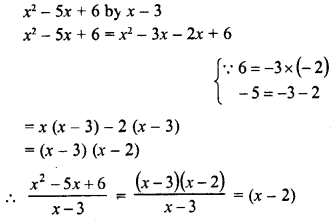Question 2.
ax2 – ay2 by ax + ay
Solution: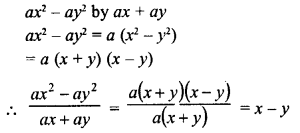Question 3.
x– y4 by x– y2
Solution:Question 4.
acx2 + (bc + ad)x + bd by (ax + b)
Solution:Question 5.
(a2 + 2ab + b2)- (a2 + 2ac + c2) by 2a + b + c
Solution: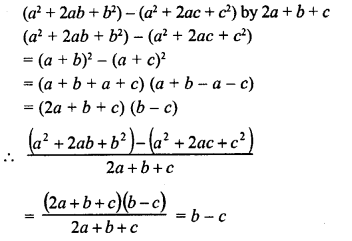Question 6.
$$\frac { 1 }{ 4 }$$ x– $$\frac { 1 }{ 2 }$$ x- 12 by $$\frac { 1 }{ 2 }$$ x – 4
Solution: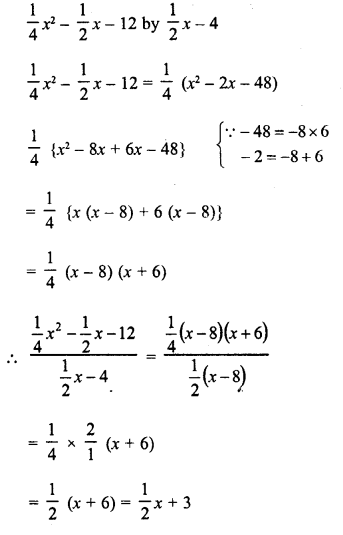Hope given RD Sharma Class 8 Solutions Chapter 8 Division of Algebraic Expressions Ex 8.6 are helpful to complete your math homework.

If you have any doubts, please comment below. Learn Insta try to provide online math tutoring for you.

## RD Sharma Class 8 Solutions Chapter 7 Factorizations Ex 7.5

These Solutions are part of RD Sharma Class 8 Solutions. Here we have given RD Sharma Class 8 Solutions Chapter 7 Factorizations Ex 7.5

Other Exercises

Factorize each of the following expressions :
Question 1.
16x2-25y2
Solution:
16x2 – 25y2 = (4x)2 – (5y)2    {∵ a2 – b2 = (a + b) (a – b)}
= (4x + 5y) (4x – 5y)

Question 2.
27x2 12y2
Solution:
27x2 – 12y2 = 3 (9x2 – 4y2)  {∵ a2 -b2 = (a + b) (a – b)}
= 3 [(3x)2 – (2y)2]
= 3 (3x + 2y) (3x – 2y)

Question 3.
144a– 289b2
Solution:
144a2 – 289b2 = (12a)2 – (17b)2    { ∵ a2b2 = (a + b) (a – b}
= (12a+ 17b) (12a- 17b)

Question 4.
12m2 – 27
Solution:
12m2 – 27 = 3 (4m2 – 9)
= 3 {(2m)2-(3)2}   {∵ a2b2 = (a + b) (a – b)}
= 3 (2m + 3) (2m – 3)

Question 5.
125x2 – 45y2
Solution:
125x2 – 45y2 = 5 (25x2 – 9y2)
= 5 {(5x-)2 – (3y)2}    {∵ a2 – b2 = (a + b) (ab}
= 5 (5x +
3y) (5x – 3y)

Question 6.
144a2 – 169b2
Solution:
144a2 – 169b2 = (12a)2 – (13b)2    {∵ a2 -b2 = (a + b) (a – b)}
= (12a + 13b) (12a-13b)

Question 7.
(2a – b)2 – 16c2
Solution:
(2a – b)2 – 16c2 = (2a – b)2 – (4c)2   {∵ a2 – b2 = (a + b) (a – b)}
= (2a – b + 4c) (2a – b – 4c)

Question 8.
(x + 2y)2 – 4 (2x -y)2
Solution:
(x + 2y)2 – 4 (2x – y)2
= (x + 2y)2 – {2 (2x –y)}2
=
(x + 2y)2 – (4x – 2y)2        {∵ a2– b2 = (a + b) (a – b)}
=
(a + 2y + 4x – 2y) (x + 2y – 4x + 2y)
= 5x (-3x + 4y)

Question 9.
3a5 – 48a3
Solution:
3a5 – 48a3 = 3a3 (a2– 16)
= 3a3 {(a)2 – (4)2}        {∵ a2 – b2 = (a + b) (a – b)}
= 3a3 (a + 4) (a – 4)

Question 10.
a4 – 16b4
Solution:
a4 – 16b4 = (a2)2 – (4b2)2
= (a2 + 4b2) (a2 – 4b2)
= (a2 + 4b2) {(a)2 – (2b)2 }   { ∵ a2 – b2 = (a + b) (a – b)}
= (a2 + 4b2) (a + 2b) (a – 2b)

Question 11.
x8 – 1
Solution:
x8 – 1 = (x4)2 – (1)2
= (x4 + 1) (x4 – 1)
= (x4+ 1) I (x2)2 – (1)2}             { a2 – b2 = (a + b) (a – b)}
= (x4 + 1) (x2 + 1) (x2 – 1)
= (x4 + 1) (x2 + 1) {(x)2 – (1)2}
= (x4+ 1)(x2 + 1)(x+ 1)(x- 1)
= (x-1)(x+ 1) (x2 + 1) (x4 + 1)

Question 12.
64 – (a + 1)2
Solution:
64 – (a + 1)2 = (8)2 – (a + 1)2    {∵ a2 – b2 = (a + b) (a – b)}
= (8 + a + 1) (8 – a – 1)
= (9 + a) (7 – a)

Question 13.
36l2 – (m + n)2
Solution:
36l2 – (m + n)2 = (6l)2 – (m + n)2        {∵  a2 – b2 = (a + b) (a – b)}
= (6l + m + n) (6l – m – n)

Question 14.
25x4y4 – 1
Solution:
25x4y4 – 1 = (5x4y4)2 – (1)2         { ∵  a2 – b2 = (a + b) (a – b)}
= (5x4y4  + 1) (5x2y2  – 1)

Question 15.Solution: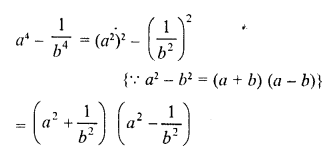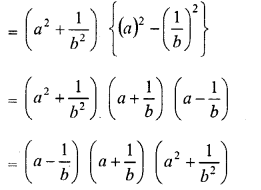Question 16.
x3 – 144x
Solution:
x3 – 144x = x (x2 – 144)
= x {(x)2 – (12)2}       { a2 – b2 = (a + b) (a – b)}
=  x (x + 12) (x – 12)

Question 17.
(x – 4y)2 – 625
Solution:
(x – 4y)2 – 625
= (x – 4y)2 – (25)2     {∵ a2 – b2 = (a + b) (a – b)}
= (x – 4y + 25) (x -4y – 25)

Question 18.
9 (a – b)2 – 100 (x -y)2
Solution:
9(a-b)2– 100(x-y)2
= {3(a-b)}2-{10(x-y)}2      { a2 – b2 = (a + b) (a – b)}
= (3a – 3b)2 – (10x – 10y)2
= (3a – 3b + 10x – 10y) (3a – 3b – 10x + 10y)

Question 19.
(3 + 2a)2 – 25a2
Solution:
(3 + 2a)2 – 25a2
= (3 + 2a)2 – (5a)2      ( a2 – b2 = (a + b) (a – b)}
= (3 + 2a + 5a) (3 + 2a – 5a)
= (3 + 7a) (3 – 3a)
= (3 + 7a) 3 (1 – a)
= 3(1-a) (3 +7a)

Question 20.
(x + y)2 – (a – b)2
Solution: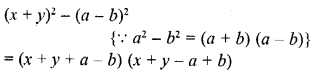Question 21.Solution: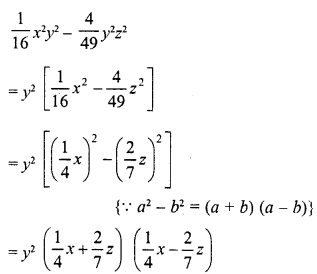Question 22.
75a3b2 – 108ab4
Solution:
75a3b2 – 108ab4
= 3ab2 (25a2 – 36b2)
= 3ab2 {(5a)2 – (6b)2}         { a2 – b2 = (a + b) (a – b)}
= 3ab2 (5a + 6b) (5a – 6b)

Question 23.
x5– 16x3
Solution:
x5 – 16x3 = x3 (x2 – 16)
= x3 {(x)2 – (4)2} { a2 – b2 = (a + b) (a – b)}
= x3 (x + 4) (x – 4)

Question 24.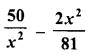Solution:Question 25.
256x5 – 81x
Solution:
256x5– 81x = x(256x4– 81)
= x {(16x2)2 – (9)2}      {∵ a2 – b2 = {a + b) (a – b)}
= x (16x2 + 9) (16x2 – 9)
= x (16x2 + 9) {(4x)2 – (3)2}
= x (16x2 + 9) (4x + 3) (4x-3)

Question 26.
a4 – (2b + c)4
Solution:
a4 – (2b + c)4
= (a2)2 – [(2b + c)2]2    { a2 – b2 = (a + b) (a – b)}
= {a2 + (2b + c)2} {a2 – (2b + c)2}
= {a2 + (2b + c)2} {(a)2 – (2b + c)2}
= {a2 + (2b + c)2} (a + 2b + c) (a -2b- c)

Question 27.
(3x + 4y)4 – x4
Solution:
(3x + 4y)4 – x4 – [(3x + 4y)2]2 – (x2)2
= [(3x + 4y)2 + x2] [(3x + 4y)2 – x2]       {∵  a2 – b2 = (a + b) (a – b)
= [(3x + 4y)2 + x2] [(3x + 4y + x) (3x + 4y – x)]
=   [(3x + 4y)2 + x2] (4x + 4y) (2x + 4y)
= [(3x + 4y)2 + x2] 4 (x + y) 2 (x + 2y)
= 8 (x + y) (x + 2y) [(3x + 4y)2 + x2]

Question 28.
p2q2 – p4q4
Solution:
p2q2– p4q4 =p2q2 (1 -p2q2)
=p2q2 [(1)2 – (pq)2]   { a2 – b2 = (a + b) (a – b)
= p2q2 (1 +pq) (1 -pq)

Question 29.
3x3y – 243xy3
Solution:
3x3y – 243xy3
= 3xy (x2 – 81y2)
= 3xy [(x)2 – (9y)2]
= 3xy (x + 9y) (x – 9y)

Question 30.
a4b4 – 16c4
Solution:
a4b4 – 16c4 = (a2b2)2 – (4c2)2
= (a2b2 + 4c2) (a2b2 – 4c2)
= (a2b2 + 4c2) [(ab)2 – (2c)2]      { a2 – b2 = (a + b) (a – b)
= (a2b2 + 4c2) (ab + 2c) (ab – 2c)

Question 31.
x4-625
Solution:
x4 – 625 = (x2)2 – (25)2   { a2 – b2 – (a + b) (a – b)
= (x2 + 25) (x2 – 25)
= (x2 + 25) [(x)2 – (5)2]
= (x2 + 25) (x + 5) (x – 5)

Question 32.
x4-1
Solution:
x4 – 1 = (x2)2 – (1)2 = (x2 + 1) (x2 – 1)
= (x2 + 1) [(x)2 – (1)2]
= (x2 + 1) (x + 1) (x – 1)

Question 33.
49 (a – b)2 -25 (a + b)2
Solution:
49 (a – by -25 (a + b)2
= [7 (a – b)]2 [5 (a + b)]2
= (7a – 7b)2 – (5a + 5b)2  { a2 – b2 = (a + b) (a – b)
= (7a -7b + 5a + 5b) (7a – 7b -5a- 5b)
=(12a – 2b)(2a – 12b)
= 2 (6a – b) 2 (a – 6b)
= 4 (6 a- b) (a – 6b)

Question 34.
x – y – x2 + y
Solution:
x-y-x2 + y2 = (x-y)-(x2-y2) {∵ a2 – b2 = (a + b) (a – b)
= {x-y)-(x + y)(x-y)
= (x-y)(1 – x – y)

Question 35.
16 (2x – 1)2 – 25y2
Solution:
16 (2x – 1)2 – 25y2
= [4 (2x – 1)]2 – (5y)2
= (8x – 4)2 – (5y)2
= (8x – 4 + 5y) (8x -4-5y)
= (8x + 5y – 4) (8x – 5y – 4)

Question 36.
4 (xy + 1)2 – 9 (x – 1)2
Solution:
4 (xy + 1)2 – 9 (x – 1)2
=
[2 (xy + 1)]2 – [3 (x – 1)]2
= (2xy + 2)2 – (3x – 3){∵ a2 – b2 = (a + b) (a – b)
= (2xy + 2 + 3x – 3) (2xy + 2 – 3x + 3)
= (2xy + 3x – 1) (2xy – 3x + 5)

Question 37.
(2x + 1)2 – 9x4
Solution:
(2x + 1)2 – 9x4 = (2x + 1)2 – (3x2)2    { a2 – b2 = (a + b) (a – b)
= (2x + 1 + 3x2) (2x + 1 – 3x2)
= (3x2 + 2x + 1) (-3x + 2x + 1)

Question 38.
x4 – (2y- 3z)2
Solution:
x4 – (2y – 3z)2 = (x2)2 – (2y – 3z)2
= (x2 + 2y- 3z) (x2 – 2y + 3z)

Question 39.
a2-b2 +a-b
Solution:
a2 – b2 + a – b
= (a + b) {a – b) + 1 (a – b)
= (a – b) (a + b + 1)

Question 40.
16a4 – b4
Solution:
16a4 – b4
= (4a2)2 – (b2)2            {   a2 – b2 = (a + b) (a – b)
= (4a2 + b2) (4a2 – b2)
= (4a2 + b2) {(2a)2 – (b)2}
= (4a2 + b2) (2a + b) (2a – b)

Question 41.
a4 – 16 (b – c)4
Solution:
a4 – 16 (b- c)4 = (a2)2 – [4 (b – c)2]{   a2 – b2 = (a + b) (a – b)
= [a2 + 4 (b – c)2] [a2 – 4 (b – c)2]
= [a2 + 4 (b – c)2] [(a)2 – [2 (b – c)]2]
= [a2 + 4 (b – c)2] [(a)2 – (2b – 2c)2]
= [a2 + 4 (b – c)2] (a + 2b – 2c) (a – 2b + 2c)

Question 42.
2a5 – 32a
Solution:
2a5 – 32a = 2a (a4 – 16)
= 2a [(a2)2 – (4)2]  {∵  a2 – b2 = (a + b) (a – b)
= 2a (a2 + 4) (a2 – 4)]
= 2a (a2 + 4) [(a)2 – (2)2]
= 2a (a2 + 4) (a + 2) (a – 2)

Question 43.
a4b4 – 81c4
Solution:
a4b4 – 81c4 = (a2b2)2 – (9c2)2
= (a2b2 + 9c2) (a2b2 – 9c2
{∵ a2 – b2 = (a + b) (a – b)
= (a2b2 + 9c2) {(ab)2 – (3c)2}
= (a2b2 + 9c2) (ab + 3c) (ab – 3c)

Question 44.
xy9-yx9
Solution:
xy9yx9 = xy (y8 – x8)
= xy [(y4)2 – (x4)2{∵  a2 – b2 = (a + b) (a – b)}
= xy(y4 + x4)(y4-x4)
= xy (y4 + x4) {(y2)2 – (x2)2}
= xy (y4 + x4) (y2 + x2) (y2 – x2)
= xy (y4 + x4) (y2 + x2) (y + x) (y – x)

Question 45.
x3 -x
Solution:
x3-x = x(x2 1)
= x [(x)2 – (1)2] = x (x + 1) (x – 1)

Question 46.
18a2x2 – 32
Solution:
18a2x2 – 32
= 2 [9a2x2 – 16]
= 2 [(3ax)2 – (4)2]   { a2 – b2 = (a + b) (a – b)
= 2 (3ax + 4) (3ax – 4)

Hope given RD Sharma Class 8 Solutions Chapter 7 Factorizations Ex 7.5 are helpful to complete your math homework.

If you have any doubts, please comment below. Learn Insta try to provide online math tutoring for you.

## RD Sharma Class 8 Solutions Chapter 8 Division of Algebraic Expressions Ex 8.5

These Solutions are part of RD Sharma Class 8 Solutions. Here we have given RD Sharma Class 8 Solutions Chapter 8 Division of Algebraic Expressions Ex 8.5

Other Exercises

Question 1.
Divide the first polynomial by the second polynomial in each of the following. Also, write the quotient and remainder.
(i) 3x2 + 4x + 5, x – 2
(ii) 10x2 – 7x + 8, 5x – 3
(iii) 5y3– 6y2 + 6y-1,5y-1
(iv)x4-x3 + 5x,x-1
(v) y4 +y2,y2-2
Solution:
(i) 3x2 + 4x + 5, x – 2
= 3x (x – 2) + 10x + 5
= 3x (x – 2) + 10 (x – 2) + 25
∴ Quotient = 3x + 10
Remainder = 25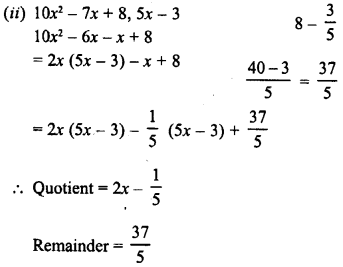(iii) 5y3 – 6y2 + 6y – 1, 5y – 1
= y(5y – 1) – 5y2 + 6y- 1
= y2 (5y – 1) -y (5y – 1) + 5y – 1
= y2 (5y- 1) -y (5y- 1) + 1 (5y- 1)
∴ Quotient = y2 – y + 1 and Remainder = 0
(iv) x4 – x3 + 5x, x – 1
= x3(x – 1) + 5x
= x3 (x – 1) + 5 (x – 1) + 5
∴ Quotient = x3 + 5, Remainder = 5
(v) y4+y2,y2– 2
= y2(y– 2) + 3y2
= y2 (y2 – 2) + 3 (y2 – 2) + 6
∴ Quotient =y2 + 3 and Remainder = 6

Question 2.
Find, whether or not the first polynomial is a factor of the second :
(i) x + 1, 2x2 + 5x + 4
(ii) y- 2, 3y3 + 5y2 + 5y + 2
(iii) 4x2 – 5, 4.x4 + 7x2 + 15
(iv) 4-z, 3z2 – 13z + 4
(v) 2a-3,10a2 – 9a – 5
(vi) 4y+1 ,8y2-2y + 1
Solution:
(i) x + 1, 2x2 + 5x + 4
2x2 + 5x + 4 = 2x (x + 1) + 3x + 4
= 2x (x + 1) + 3 (x + 1) + 1
∵ Remainder = 1
∴ x + 1 is not a factor of 2x2 + 5x + 4
(ii) y – 2, 3y3 + 5y2 + 5y + 2
3y3 + 5y2 + 5y + 2 = 3y2(y – 2)+11y2 + 5y + 2
= 3y2(y – 2)+11y (y – 2) + 27y + 2
= 3y2 (y – 2) + 11y (y – 2) + 27 (y – 2) + 56
∵ Remainder = 56
∴ y – 2 is not a factor of 3y3 + 5y2 + 5y + 2
(iii) 4x2 – 5, 4x4 + 7x2 + 15
4x4 + 7x2 + 15 = x2 (4x2 – 5) + 12x2 + 15
= x2 (4x2 – 5) + 3 (4x2 – 5) + 30
∵ Remainder = 30
∴ 4x2 – 5 is not a factor of 4x4 + 7x2 + 15
(iv) 4 – z, 3z2 – 13z + 4
3z2 – 13z + 4 = -3z (-z + 4) – z + 4
= -3z (-z + 4) + 1 (-z + 4)
∵ Remainder = 0
∴ 4 – z or – z + 4 is a factor of 3z2 – 13z + 4
(v) 2a – 3, 10a2 – 9a – 5
10a2 – 9a – 5 = 5a (2a – 3) + 6a – 5
= 5a (2a – 3) + 3 (2a – 3) + 4
∵ Remainder = 4
∴ 2a – 3 is not a factor of 10a2 – 9a – 5
(vi) 4y + 1, 8y2 – 2y + 1
8y2 – 2y + 1 = 2y (4y + 1) – 4y + 1
= 2y (4y + 1) – 1 (4y + 1) + 2
∵ Remainder = 2
∴ 4y + 1 is not a factor of 8y2 – 2y + 1

Hope given RD Sharma Class 8 Solutions Chapter 8 Division of Algebraic Expressions Ex 8.5 are helpful to complete your math homework.

If you have any doubts, please comment below. Learn Insta try to provide online math tutoring for you.

## RD Sharma Class 8 Solutions Chapter 8 Division of Algebraic Expressions Ex 8.3

These Solutions are part of RD Sharma Class 8 Solutions. Here we have given RD Sharma Class 8 Solutions Chapter 8 Division of Algebraic Expressions Ex 8.3

Other Exercises

Question 1.
x+2x2+3x4-x5 by 2x
Solution: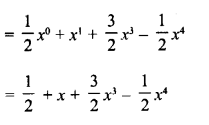Question 2.
y4-3y3+ $$\frac { 1 }{ 2 }$$ y2 by 3y
Solution: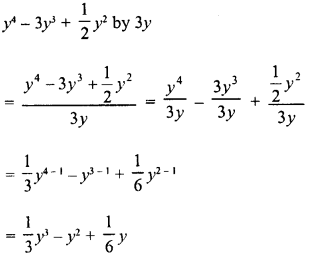Question 3.
-4a3 + 4a2 + a by 2a
Solution: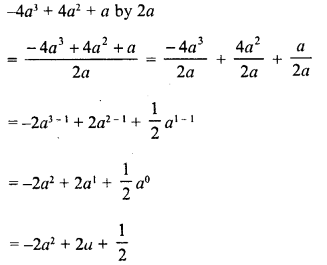Question 4.
-x6 + 2x4 + 4.x3 + 2x2 by √2x2
Solution: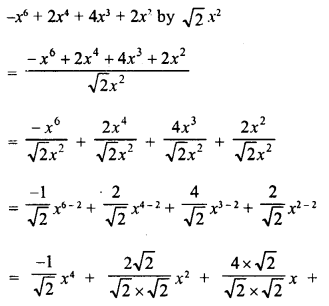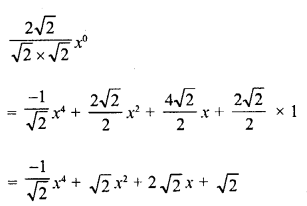Question 5.
5z3 – 6z2 + 7z by 2z
Solution:Question 6.
√3 a4 + 2 √3 a3 + 3a2 – 6a by 3a
Solution:Hope given RD Sharma Class 8 Solutions Chapter 8 Division of Algebraic Expressions Ex 8.3 are helpful to complete your math homework.

If you have any doubts, please comment below. Learn Insta try to provide online math tutoring for you.

## RD Sharma Class 8 Solutions Chapter 7 Factorizations Ex 7.6

These Solutions are part of RD Sharma Class 8 Solutions. Here we have given RD Sharma Class 8 Solutions Chapter 7 Factorizations Ex 7.6

Other Exercises

Factorize each of the following algebraic expressions :
Question 1.
4x2 + 12xy + 9y2
Solution:
4x2 + 12xy + 9y2 = (2x)2 + 2 x 2x x 3y + (3y)2 {∵ a2 + 2ab + b2 = (a +b)2}
= (2x + 3y)2

Question 2.
9a2 – 24ab + 16b2
Solution:
9a2 – 24ab + 16b2
= (3a)2 – 2 x 3a x 4b + (4b)2     {∵ a2 – 2ab + b2 = (a – b)2}
= (3a – 4b)2

Question 3.
36a2 – 6pqr + 9r2
Solution:
p2q2 – 6pqr + 9r2
= (pq)2 – 2 x pq x3r + (3r){∵ a2 – 2ab + b2 = (a -b)2}
= (pq-3r)2

Question 4.
36a2 + 36a + 9
Solution:
36a2 + 36a + 9
= (6a)2 + 2 x 6a x 3 + (3)2   {∵ a2 + 2ab + b2 = (a + b)2
= (6a + 3)2

Question 5.
a2 + 2ab + b2 – 16
Solution:
a2 + 2ab + b2 – 16
= (a + b)2 – (4)2     {∵ a2 + 2ab + b2 = (a + b)2 and a2 – b2 = (a + b) (a – b)}
= (a + b + 4) (a + b – 4)

Question 6.
9z2 – x2 + 4xy – 4y2
Solution:
9z2 – x2 + 4xy – 4y2    {∵ a2 – b2 = (a + b) (a – b) and a2 – 2ab + b2 (a – b)2}
= 9z2 – (x2 – 4xy + 4y2)
= (3z)2 – [(x)2 – 2 x x x 2y + (2y)2]
= (3z)2-(x-2y)2
= (3z + x – 2y) (3z – x + 2y)

Question 7.
9a4 – 24a2b2 + 16b4 – 256
Solution: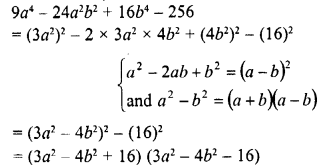Question 8.
16 – a6 + 4a3b3 – 4b6
Solution: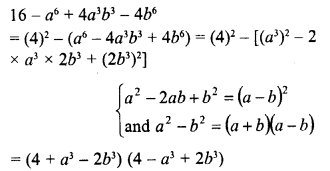Question 9.
a2 – 2ab + b2 – c2
Solution: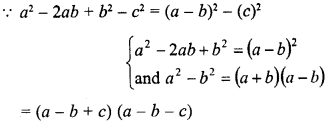Question 10.
x2 + 2x + 1 – 9y2
Solution:Question 11.
a2 + 4ab + 3b2
Solution:
a2 + 4ab + 3b2
= a2 + 4ab+ 4b2 – b2
= (a)2 + 2 x a x 2b + (2b)2 – b(∵ 3b2 = 4b2 – b2)
= (a + 2b)2 – (b)2   {∵ a2 – b2 = (a +b) (a – b)}
= (a + 2b + b) (a + 2b- b)

Question 12.
96 – 4x-x2
Solution:
96 – 4x – x2 = 96 – (4x + x2)
= 96 – [(x)2 + 2 x x x 2 + (2)2] + (2)2   (on completing the square)
= 96 + 4 – (x + 2)2 = 100 – (x + 2)2
= (10)2 – (x + 2)2
= (10 + x + 2) (10 – x- 2)
= (x + 12) (-x + 8)

Question 13.
a4 + 3a2 + 4
Solution:
a4 + 3a2 + 4
= (a2)2 + (2)2 + 2 x a2 x 2 – a2   (on completing the square)
= (a2 + 2)2 – (a)2
= (a2 + 2 + a) (a2 + 2 – a)
= (a1 + a + 2) (a2 – a + 2)

Question 14.
4a4 + 1
Solution:
4x4 + 1 = (2a2)2 + (1)2 + 2 x 2x2 x 1 – 2 x 2xx 1  (completing the square)
= (2x2 + 1)2 – 4a2
= (2x2 + 1)2 – (2a)2   {a2 – b2 = (a + b) (a – b)}
= (2x2 + 1 + 2a) (2a2 + 1 – 2a)
= (2a2 + 2a + 1) (2a2 – 2a + 1)

Question 15.
4x4+y4
Solution:
4a4 + y4 = (2x2)2 + (y2)2 + 2 x 2x2y2 – 2 x 2x2y2
= (2x2 + y2)2 – 4x2y2
= (2x2 + y2)2 – (2xy)2
= (2x2 + y2 + 2xy) (2x2 + y2 – 2xy)
= (2x2 + 2xy + y2) (2x2 – 2xy + y2)

Question 16.
(x+ 2)2 – 6 (a + 2) + 9
Solution:
(x + 2)2 – 6 (x + 2) + 9
=  (x + 2)2 – 2 x (x + 2) x 3 + (3)2
= (x + 2 – 3)2
= (x-1)2 = (x-1)(x-1)

Question 17.
25 – p2 – q2 – 2pq
Solution: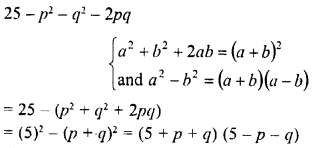Question 18.
a2 + 9y2 – 6xy – 25a2
Solution:
a2 + 9y2 – 6xy – 25a2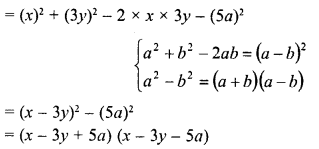Question 19.
49 – a2 + 8ab – 16b2
Solution:Question 20.
a2 – 8ab + 16b2 – 25c2
Solution: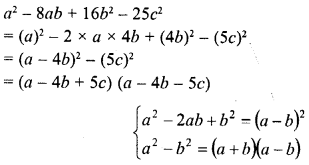Question 21.
x2 -y2+ 6y- 9
Solution: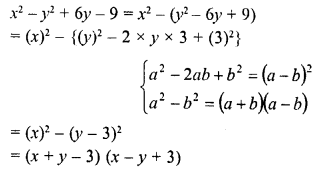Question 22.
25x2 – 10x + 1 – 36y2
Solution: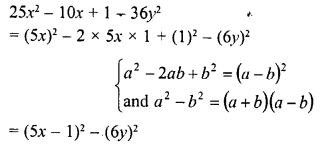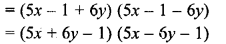Question 23.
a2-b2 + 2bc – c2
Solution: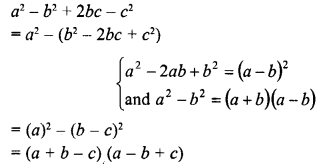Question 24.
a2 + 2ab + b2 -c2
Solution: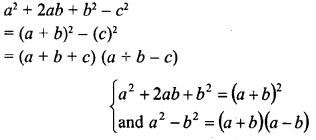Question 25.
49 -x2 – y2 + 2xy
Solution: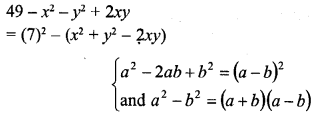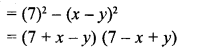Question 26.
a2 + 4b24ab 4c2
Solution: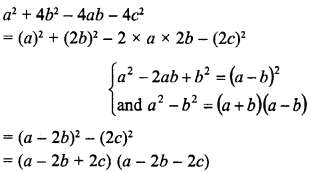Question 27.
x2 -y24xz + 4z2
Solution: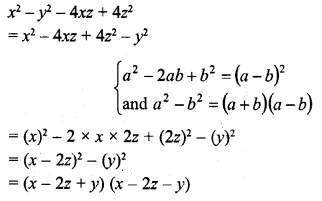Hope given RD Sharma Class 8 Solutions Chapter 7 Factorizations Ex 7.6 are helpful to complete your math homework.

If you have any doubts, please comment below. Learn Insta try to provide online math tutoring for you.

## RD Sharma Class 8 Solutions Chapter 8 Division of Algebraic Expressions Ex 8.2

These Solutions are part of RD Sharma Class 8 Solutions. Here we have given RD Sharma Class 8 Solutions Chapter 8 Division of Algebraic Expressions Ex 8.2

Other Exercises

Divide :

Question 1.
6x3y2z2 by 3x2yz
Solution: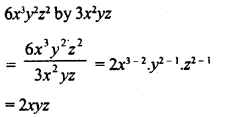Question 2.
15m2nby 5m2n2
Solution: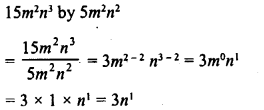Question 3.
24a3bby -8ab
Solution: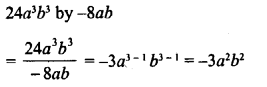Question 4.
-21abc2 by 7abc
Solution:Question 5.
72xyz2 by – 9xz
Solution:Question 6.
-72a4b5cby – 9a2b2c3
Solution: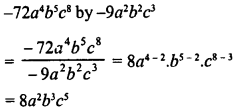Simplify :

Question 7.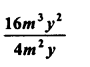Solution: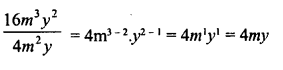Question 8.Solution: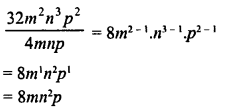Hope given RD Sharma Class 8 Solutions Chapter 8 Division of Algebraic Expressions Ex 8.2 are helpful to complete your math homework.

If you have any doubts, please comment below. Learn Insta try to provide online math tutoring for you.

## RD Sharma Class 8 Solutions Chapter 8 Division of Algebraic Expressions Ex 8.1

These Solutions are part of RD Sharma Class 8 Solutions. Here we have given RD Sharma Class 8 Solutions Chapter 8 Division of Algebraic Expressions Ex 8.1

Other Exercises

Question 1.
Write the degree of each of the following polynomials :
(i) 2x3+5x2-7
(ii) 5x2
3x +7 ’
(iii) 2x + x2
-8
(iv) $$\frac { 1 }{ 2 }$$
y7 -12y5 + 48y6 – 10
(v) 3x3 + 1

(vi) 5
(vii) 20x3 + 12x2y2– 10y2 + 20
Solution:
(i) 2x3 + 5x2-7: The degree of this polynomial is 3.
(ii) 5x2 – 3x + 2 : The degree of this polynomial is 2.
(iii) 2x + x2 – 8 : The degree of this polynomial is 2.
(iv) $$\frac { 1 }{ 2 }$$ y7 – 12y6 + 48y5 – 10 : The degree of this polynomial is 7.
(v) 3x3 + 1 : The degree of this polynomial is 3.
(vi) 5 : The degree of this polynomial is 0 as it is only constant term
(vii) 20x3 + 12x2y2 – 10y2 + 20: The degree of this polynomial is 2 + 2 = 4.

Question 2.
Which of the following expressions are not polynomials :
(i) x2 + 2x2
(ii) √a x + x2-x3
(iii) 3y3 – √5y + 9

(iv) ax1/2 + ax + 9x2 + 4
(v) 3x2 + 2x-1 + 4x + 5
Solution:
(i) x2 + 2x-2 = x2 + 2x $$\frac { 1 }{ { x }^{ 2 } }$$ =x2 + $$\frac { 1 }{ { x }^{ 2 } }$$
: It is not xx polynomial as it has negative integral power.
(ii) √ax + x2 – x3: It is polynomial.
(iii) 3y3  √5y + 9 : It is a polynomial.
(iv) ax1/2+ ax + 9x2 + 4: It is not a polynomial as  the degree of $$\frac { 1 }{ { x }^{ 2 } }$$ is an integer.
(v) 3x2 + 2x-1 + 4x + 5 : It is not a polynomial as the degree of x2, x-1 are negative.

Question 3.
Write each of the following polynomials in the standard form. Also write their degree.
(i) x2 + 3 + 6x + 5x4
(ii) a1 + 4 + 5a6
(iii) (x3 – 1) (x3 – 4)

(iv) (y3 – 2) (y3 + 11)
(v) $$\left( { a }^{ 3 }-\frac { 3 }{ 8 } \right)$$ $$\left( { a }^{ 3 }-\frac { 16 }{ 17 } \right)$$
(vi) $$\left( { a }+\frac { 3 }{ 4 } \right)$$ $$\left( { a }+\frac { 3 }{ 4 } \right)$$
Solution:
Polynomial in standard form is the polynomial in ascending order or descending order.Hope given RD Sharma Class 8 Solutions Chapter 8 Division of Algebraic Expressions Ex 8.1 are helpful to complete your math homework.

If you have any doubts, please comment below. Learn Insta try to provide online math tutoring for you.

## RD Sharma Class 8 Solutions Chapter 7 Factorizations Ex 7.7

These Solutions are part of RD Sharma Class 8 Solutions. Here we have given RD Sharma Class 8 Solutions Chapter 7 Factorizations Ex 7.7

Other Exercises

Factorize each of the following algebraic expressions :
Question 1.
x2 + 12x – 45
Solution: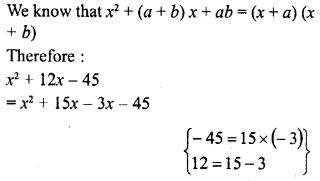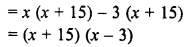Question 2.
40 + 3x – x2
Solution: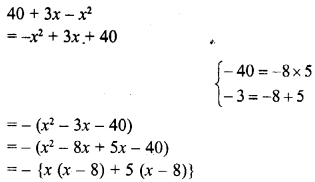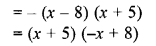Question 3.
a2 + 3a-88
Solution: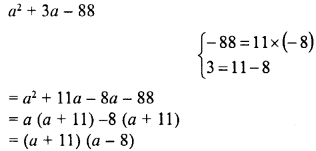Question 4.
a2 – 14a – 51
Solution: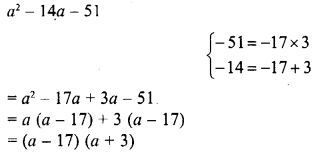Question 5.
x2 + 14x + 45
Solution:Question 6.
x2 – 22x + 120
Solution:Question 7.
x2– 11x – 42
Solution: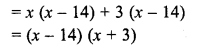Question 8.
a2 + 2a – 3
Solution:Question 9.
a2 + 14a + 48
Solution:Question 10.
x2 – 4x – 21
Solution: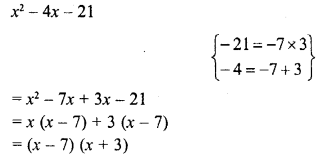Question 11.
y2 – 5y-36
Solution: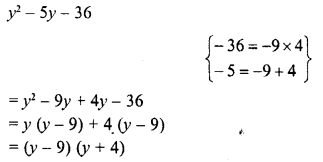Question 12.
(a2-5a)2-36
Solution: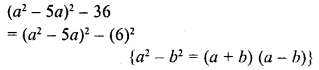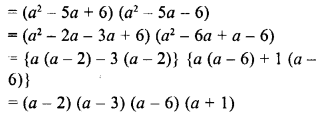Question 13.
(a + 7) (a – 10) + 16
Solution: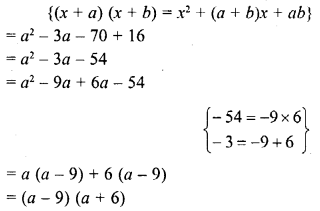Hope given RD Sharma Class 8 Solutions Chapter 7 Factorizations Ex 7.7 are helpful to complete your math homework.

If you have any doubts, please comment below. Learn Insta try to provide online math tutoring for you.

## RD Sharma Class 8 Solutions Chapter 7 Factorizations Ex 7.8

These Solutions are part of RD Sharma Class 8 Solutions. Here we have given RD Sharma Class 8 Solutions Chapter 7 Factorizations Ex 7.8

Other Exercises

Resolve each of the following quadratic trinomials into factors :
Question 1.
2x2 + 5x + 3
Solution: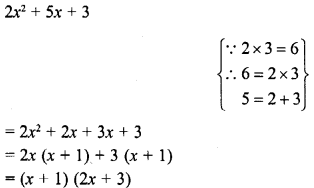Question 2.
2x2– 3x – 2
Solution: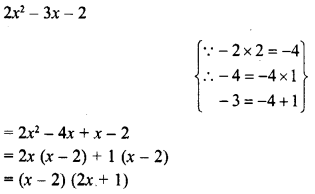Question 3.
3x2 + 10x + 3
Solution: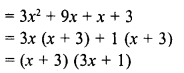Question 4.
7x – 6 – 2x2
Solution:Question 5.
7x2 – 19x – 6
Solution: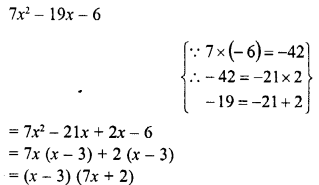Question 6.
28-31x -5x2
Solution: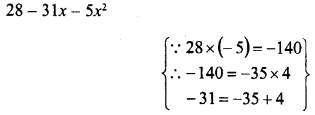Question 7.
3 + 23y – 8y2
Solution: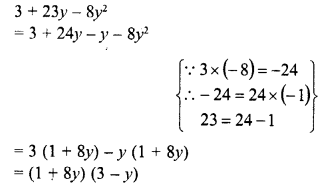Question 8.
11x2 – 54x + 63
Solution: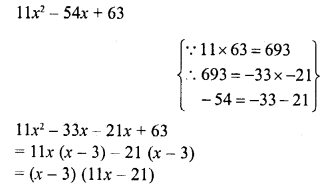Question 9.
7x-6x2 + 20
Solution:Question 10.
3x2 + 22x + 35
Solution: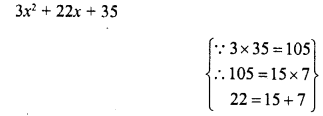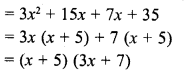Question 11.
12x2 – 17xy + 6y2
Solution: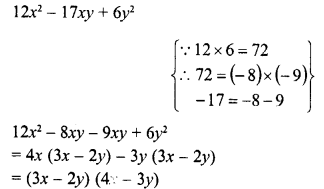Question 12.
6x2 – 5xy – 6y2
Solution: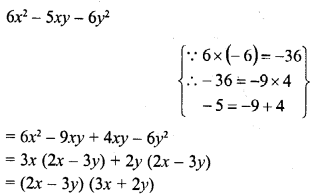Question 13.
6x2 + 13xy + 2y2
Solution:Question 14.
14x2 + 11xy – 15y2
Solution:Question 15.
6a2 + 17ab – 3b2
Solution: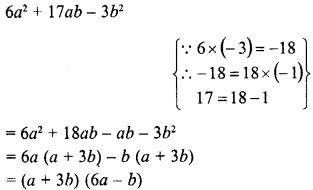Question 16.
36a2 + 12abc – 15b2c2
Solution: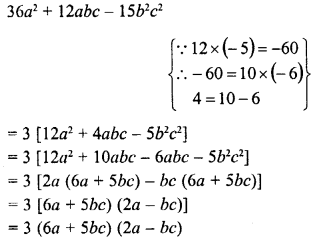Question 17.
15x2 – 16xyz – 15y2z2
Solution: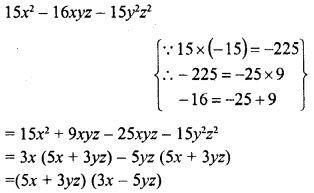Question 18.
(x – 2y)2 -5 (x- 2y) + 6
Solution:Question 19.
(2a – b)2 + 2 (2a – b) – 8
Solution:Hope given RD Sharma Class 8 Solutions Chapter 7 Factorizations Ex 7.8 are helpful to complete your math homework.

If you have any doubts, please comment below. Learn Insta try to provide online math tutoring for you.

## RD Sharma Class 8 Solutions Chapter 7 Factorization Ex 7.3

These Solutions are part of RD Sharma Class 8 Solutions. Here we have given RD Sharma Class 8 Solutions Chapter 7 Factorizations Ex 7.3

Other Exercises

Factorize each of the following algebraic expressions.

Question 1.
6x (2x – y) + 7y (2x – y)
Solution:
6x (2x – y) + 7y (2x – y)
= (2x – y) (6x + 7y)
[∵ (2x – y) is common]

Question 2.
2r (y – x) + s (x – y)
Solution:
2r (y – x) + s (x – y)
-2r (x – y) +s (x – y)
= (x – y) (-2r + s)                   [(x – y) is common]
= (x-y) (s-2r)

Question 3.
7a (2x – 3) + 2b (2x – 3)
Solution:
7a (2x – 3) + 3b (2x – 3)
= (2x – 3) (7a + 3b)               [(2x – 3) is common]

Question 4.
9a (6a – 5b) – 12a2 (6a – 5b)
Solution:
9a (6a – 5b) – 12a2 (6a – 5b)
HCF of 9 and 12 = 3
∴ 3a (6a – 5b) (3 – 4a)
{(6a – 5b) is common}

Question 5.
5 (x – 2y)2 + 3 (x – 2y)
Solution:
5 (x – 2y)2 + 3 (x – 2y)
= 5 (x – 2y) (x – 2y) + 3 (x – 2y)
= (x – 2y) {5 (x – 2y) + 3}
{(x – 2y) is common}
= (x – 2y) (5x – 10y + 3)

Question 6.
16 (2l – 3m)2 – 12 (3m – 2l)
Solution:
16 (2l – 3m)2 – 12 (3m-2l)
= 16 (2l – 3m) (2l – 3m) + 12 (2l – 3m)
HCF of 16, 12 = 4 4 (2l-3m) {4 (2l- 3m) + 3}
{(2l – 3m) is common}
= 4 (2l -3m) (8l- 12m+ 3)

Question 7.
3a (x – 2y) – b (x – 2y)
Solution:
3a (x – 2y) – b (x – 2y)
= (x – 2y) (3a – b)
{(x – 2y) is common}

Question 8.
a2 (x + y) + b2 (x + y) + c2 (x + y)
Solution:
a2 (x + y) + b2 (x + y) + c2 (x + 3’)
= (x + y) (a2 + b2 + c2)
{(x + y) is common}

Question 9.
(x-y)2 + (x -y)
Solution:
(x – y)2 + (x- y) = (x – y) (x – y) + (x – y)
= (x – y) (x – y + 1)                          {(a – y) is common}

Question 10.
6 (a + 2b) – 4 (a + 2b)2
Solution:
6 (a + 2b) – 4 (a + 2b)2
= 6 (a + 2b) – 4 (a + 2b) (a + 2b)
HCF of 6, 4 = 2
= 2 {a + 2b) {3 – 2 {a + 2b)
{2 (a + b) is common}
= 2 (a + 2b) (3-2 a- 4b)

Question 11.
a (x -y) + 2b (y – x) + c (x -y)2
Solution:
a (x -y) + 2b (y – x) + c (x -y)2
= a (x – y) – 2b (x – y) + c (x – y) {x – y)
= (x – y) {x – 2b + c (x – y)}
{(a – y) is common}
= (a – y) (a – 2b + cx – cy)

Question 12.
– 4 (a – 2y)2 + 8 (a – 2y)
Solution:
– 4 (x – 2y)2 + 8 (x – 2y)
= – 4 (x – 2y) (x – 2y) + 8 (x – 2y)
{- 4 (x – 2y) is common}
= – 4 (x – 2y) (x – 2y – 2)
= 4 (x – 2y) (2 – x + 2y)

Question 13.
x3 (a – 2b) + a2 (a – 2b)
Solution:
x3 (a – 2b) + x2 (a – 2b)
HCF of x3, x2 = x2
∴
x2 (a – 2b) (x + 1)
{x2 (x – 2b) is common}
= x2 (x – 2b) (x + 1)

Question 14.
(2x – 3y) (a + b) + (3x – 2y) (a + b)
Solution:
(2x – 3y) (a + b) + (3x – 2y) (a + b)
= (a + b) {2x – 3y + 3x – 2y}
{(x + b) is common}
= (a + b) (5x – 5y)
= 5 (a + b) (x – y)

Question 15.
4 (x + y) (3a – b) + 6 (a + y) (2b – 3a)
Solution:
4 (x + y) (3a – b) + 6 (a + y) (2b – 3a)
= 4 (x + y) (3a – b) – 6 (x + y) (3a – 2b)
HCF of 4, 6 = 2
= 2 (x + y) {2 (3a – b) – 3 (3a – 2b)}
= 2 (x + 3) {6a – 2b – 9a + 6b}
= 2 (x +y) {-3a + 4b}
= 2 (x + y) (4b – 3a)

Hope given RD Sharma Class 8 Solutions Chapter 7 Factorizations Ex 7.3 are helpful to complete your math homework.

If you have any doubts, please comment below. Learn Insta try to provide online math tutoring for you.

## RD Sharma Class 8 Solutions Chapter 7 Factorizations Ex 7.9

These Solutions are part of RD Sharma Class 8 Solutions. Here we have given RD Sharma Class 8 Solutions Chapter 7 Factorizations Ex 7.9

Other Exercises

Factorize each of the following quadratic polynomials by using the method of completing the square.
Question 1.
p2 + 6p + 8
Solution:
p2 + 6p + 8
= p2 + 2 x p x 3 + 32 – 32 + 8   (completing the square)
= (p2 + 6p + 32) – 1
= (p + 3)2 – 12
= (P + 3)2 – (1)2       { ∵ a2 + b2  = (a+b) (a-b)}
= (p +3+1) (p + 3 -1)
= (p+4) (p+ 2)

Question 2.
q2 – 10q + 21
Solution:
q2 – 10q + 21
= (q)2 – 2 x q x 5 + (5)2 – (5)2 + 21   (completing the square)
= (q)2 – 2 x q x 5 + (5)2 -25+21
= (q)2-2 x q x 5 + (5)2 – 25 +21
= (q)2-2 x q x 5 + (5)2 – 4
= (q – 5)2 – (2)     {∵ a2 – b2 = (a + b) (a – b)}
= (q- 5 + 2) (q-5-2)
=(q- 3) (q-7)

Question 3.
4y2 + 12y + 5
Solution:
4y+12y + 5
= (2y)2 + 2 x 2y x 3 + (3)2 – (3)2 + 5    (completing the square)
= (2y + 3)2 – 9 + 5
= (2y + 3)2 – 4
= (2y + 3)2-(2)2   {∵ a2 – b2 = (a + b) (a – b)}
= (2y + 3 + 2) (2y + 3 – 2)
= (2y + 5) (2y+ 1)

Question 4.
p2 + 6p- 16
Solution:
p2 + 6p – 16
= (p)2 + 2 x  p x 3 + (3)2 – (3)2 – 16    (completing the square)
= (p)2 + 2 x p x 3 + (3)2 – 9 – 16
= (p + 3)2 – 25
= (p + 3)2 – (5)2     {∵ a2 -b2 = {a + b) (a – b)}
= (p + 3 + 5)(p + 3-5)
= (p + 8) (p – 2)

Question 5.
x2 + 12x + 20
Solution:
x2 + 12x + 20
= (x)2 + 2 x x x 6 + (6)2 – (6)2 + 20   (completing the square)
= (x)2 + 2 x x x6 + (6)2 -36 + 20
= (x + 6)2 -16
= (x + 6)2 – (4)2   {∵ a2 – b2 = (a + b) (a – b)}
= (x + 6 + 4) (x + 6 – 4)
= (x + 10) (x + 2)

Question 6.
a2 – 14a – 51
Solution:
a2 – 14a-51
= (a)2 – 2 x x 7 + (7)2 – (7)2 – 51       (completing the square)
= (a)2 – 2 x a x 7 + (7)2 – 49 – 51
= (a – 7)2 – 100
= (a – 7)2 – (10)2    {∵  a2 – b2 = (a + b) (a – b)}
= (a – 7 + 10) (a – 7 – 10)
= (a + 3) (a – 17)

Question 7.
a2 + 2a – 3
Solution:
a2 + 2a – 3
= (a)2 + 2 x a x 1 + (1)2 – (1)2 – 3   (completing the square)
= (a)2 + 2 x a x 1 + (1)2 – 1 – 3
= (a + 1)2 – 4
= (a + 1)2 – (2){∵ a2 – b2 = (a + b) (a – b)}
= (a + 1 + 2) (a + 1 – 2)
= (a + 3) (a – 1)

Question 8.
4x2 – 12x + 5
Solution:
4x2 – 12x + 5
= (2x)2 – 2 x 2x x 3 + (3)2 – (3)2 + 5  (completing the square)
= (2x)2 – 2 x 2x x 3 + (3)2 -9 + 5
= (2x – 3)2 – 4
= (2x – 3)2 – (2)2
{∵ a2b2 = (a + b) (a – b)}
=
(2x – 3 + 2) (2x – 3 – 2)
= (2x – 1) (2x – 5)

Question 9.
y2 – 7y + 12
Solution: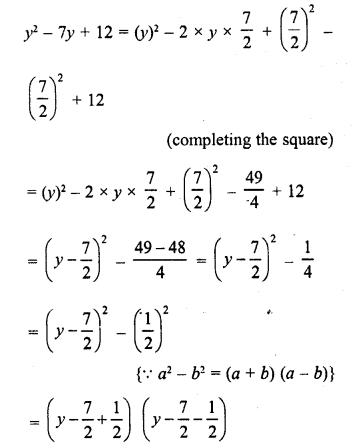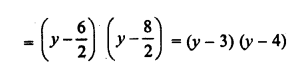Question 10.
z2-4z-12
Solution:
z2 – 4z – 12
= (z)2 – 2 z x 2 + (2)2 – (2)2 – 12  (completing the square)
= (z)2 – 2 x z x 2 + (2)2 – 4 – 12
= (z-2)2-16
= (z-2)2-(4)2   {∵ a2 – b2 = (a + b) (a – b)}
= (z – 2 + 4) (z – 2 – 4)
= (z + 2)(z-6)

Hope given RD Sharma Class 8 Solutions Chapter 7 Factorizations Ex 7.9 are helpful to complete your math homework.

If you have any doubts, please comment below. Learn Insta try to provide online math tutoring for you.